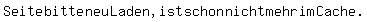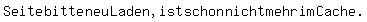# Joule-Thomson-Coefficient (JTC)

Mike1977
Hi from Germany,

I would like to calculate the JTC for a concrete example.

From a pressure vessel full (in german we say "Druckbehälter" (db)) with dry air (Vdb=100 m3; pdb=300 bar; Tdb=293,13 K) flows out the air after the valve outside (pamb=1 bar). I suppose that Tdb and pb in the pressure vessel remain constant.

With the following approach I would like to calculate the cooling of the air. The constants a, b and R are known to me.:What values I have to use for T and cp?

I think, I have to use the values from the pressure vessel, to calculate the temperature of the air after throttling to atmospheric pressure. Therfore I have to calculate the molar isobaric heat capacity of air cp,db in the pressure vessel (at Tdb = 293.13 K and pdb = 300 bar).

Am I right ??Mentor
The pressure change is too large to use the JT coefficient, since JT is just the derivative of temperature with respect to pressure at constant enthalpy. If you are trying to calculate the temperature coming out of the throttle valve for your situation, then the easiest way to do this is by using air tables. Do you have tables that give enthalpy of air as a function of temperature and pressure? The key characteristic of an adiabatic throttle valve is that the change in enthalpy is equal to zero. So you need to find the enthalpy at the initial conditions, and then, for the same enthalpy, find the temperature corresponding to the final pressure.

Chet

•Mike1977
Mike1977

my example was a fictive one to understand the general thermodynamic equations. But if you say that I can not use JT coefficient to calculate the cooling, why different literature reference gives JT coefficient for very high pressures. Furthermore the literature references shows no scope of application regarding the pressures.

However, my general question remains: If I want to calculate the JTC for two pairs of pressure (for example 30 bar and 1 bar), what values do I use for the molar isobaric Wärmekapzität c_p and the temperature T (the values of higher or lower the pressure)?

http://webbook.nist.gov/cgi/fluid.c...nit=kJ/mol&WUnit=m/s&VisUnit=uPa*s&STUnit=N/m

Thank you for your help, Mike

Mike1977
Hi Chat,

habe you an idea, where I can find those tables? the tables that I had found, base on the assumption of an ideal gas. But the air in the pressure vessel can not discribe by the ideal gas theory.

Thank you and beste regards, Mike

Mentor
Try the tables at the end of this reference: http://www.nist.gov/data/PDFfiles/jpcrd581.pdf [Broken]

I merely Googled "Thermodynamic Properties for Air"

Last edited by a moderator:
Mike1977
Thank you Chet for the link. Have you an idea regarding the 3rd post in this thread? Especially the fact, for which conditions I have to use the heat capacity (for the high pressure or the low pressure). Because I want to compare the solutions of:
JTC vs. enthalpy-tables.

Best regards, Michael

Mentor
Thank you Chet for the link. Have you an idea regarding the 3rd post in this thread? Especially the fact, for which conditions I have to use the heat capacity (for the high pressure or the low pressure). Because I want to compare the solutions of:
JTC vs. enthalpy-tables.

Best regards, Michael
This is going to be a difficult comparison to make because the pressure difference is so huge, and the JT equation is only valid for determining the derivative of the temperature with respect to pressure at the starting conditions. If there is any curvature to the relationship between temperature and pressure at constant enthalpy, the answer using JT is going to be way off. I would first try it using the parameter values at the initial pressure and temperature. This would give you the predicted temperature using Euler's method. I might also try to evaluate the JT coefficient using the geometric mean pressure and see how that compares. As another alternative, you could apply the JT equation over a sequence of pressure intervals, like a numerical integration and sum up the temperature changes. As the pressure intervals become smaller, the answer should approach the result obtained using the tables. So, there are many things you could try, but the least accurate is going to what you obtain if you use the JT equation once at the initial conditions.

Chet